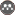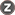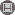year 3, Issue 4 (Winter 2014 2014)                   E.E.R. 2014, 3(4): 58-68 | Back to browse issues page

BibTeX | RIS | EndNote | Medlars | ProCite | Reference Manager | RefWorks
Send citation to:Classification of three formulae Lane_Kalinske, Bagnold, Toffaleti in Soolegan River using AHP. E.E.R. . 2014; 3 (4) :58-68
URL: http://magazine.hormozgan.ac.ir/article-1-200-en.html
Abstract:   (3204 Views)

For selecting the most proper equation of sediment estimation discharge, there is a great need to evaluate them for the concerned river. In this paper suspended sediment estimation formulae in Soolegan River using Analytical Hierarchy Processing (AHP) is investigated. Three equations of sediment estimation Lane_Kalinske, Bagnold, Toffaleti were applied in this study. For estimating the amount of suspended sediment discharge for Soolegan River, numerical methods were used. After this step the amount of the accuracy of each of the formulae were evaluated with three coefficients (R2, NS, Dv). In addition, the cost of solving each of the equation and also the numbers of the input parameters to each of them were used for classifying these three formulae. At first couple evaluation matrix for each of the criteria was created and then at last by calculating the final weight for each of the equations the preference of them was determined. Toffaleti with final weight of 0.39, Bagnold with 0.33 and Lane_Kalinske with 0.31 were classified in three groups for estimating the suspended sediment discharge.